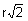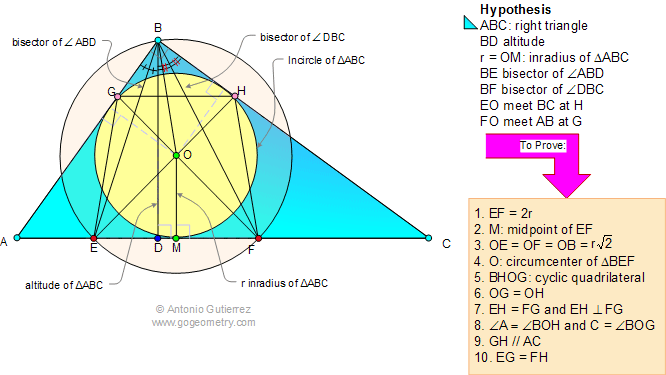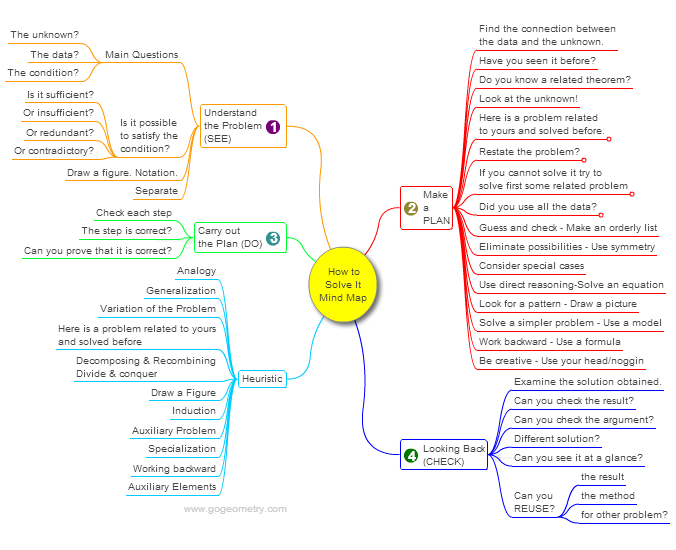# Geometry Problem 29 with Solution: Right triangle, Altitude, Angle Bisector, Incenters, Inradius, Perpendicular Lines, Cyclic Quadrilateral, Circumcenter, Parallel Lines, 45 Degrees, Congruence.

< PREVIOUS PROBLEM  |  NEXT PROBLEM >

In triangle ABC, angle B is a right angle, BD is the altitude, O is the incenter, r is the inradius, BE is the bisector of angle ABD, BF is the bisector of angle DBC. EO and FO are extended until they meet sides BC and AB at H and G, respectively. Prove the following:

1. EF = 2r

2. M is the midpoint of EF

3. OE = OF = OB =4. O is the circumcenter of triangle BEF

6. OG = OH

7.EH and FG are congruent and perpendicular

8. Angles A and BOH are congruent, similarly C and BOG

9. GH is parallel to AC

10. EG = FH

.Hints: See Geometry Help

## Mind Map: George Pólya's 1945 book, "How to Solve It"

George Pólya's 1945 book "How to Solve It, A new aspect of Mathematical Method", is a book describing methods of problem solving. It suggests the following steps when solving a mathematical problem: (1) First, you have to understand the problem. (2) After understanding, then make a plan. (3) Carry out the plan. (4) Look back on your work. How could it be better?
A mind map is a diagram used to visually organize information, knowledge, concepts or ideas.Home | Geometry | Search | Problems | 21-30 | Altitude | Circle Tangent Line | Right triangle, Altitude and Incircle | Email | By Antonio Gutierrez
Post or view a solution to the problem 29

Last updated: Sep 19, 2014